# Linear Regression for Machine Learning

By  Shaurya Singh    26 - 28 July, 20Share article on social media

What is Linear Regression?

Before learning about Linear Regression we need to understand what Regression is. Regression is a statistical method to used for estimating relationships between a dependent variable and one or more independent variables. That is, regression helps us to model the cause and effect of one or many independent variables on a single variable let's say 'y', and since the value of 'y' is dependent on these set of independent variables it is known as a dependent  variable.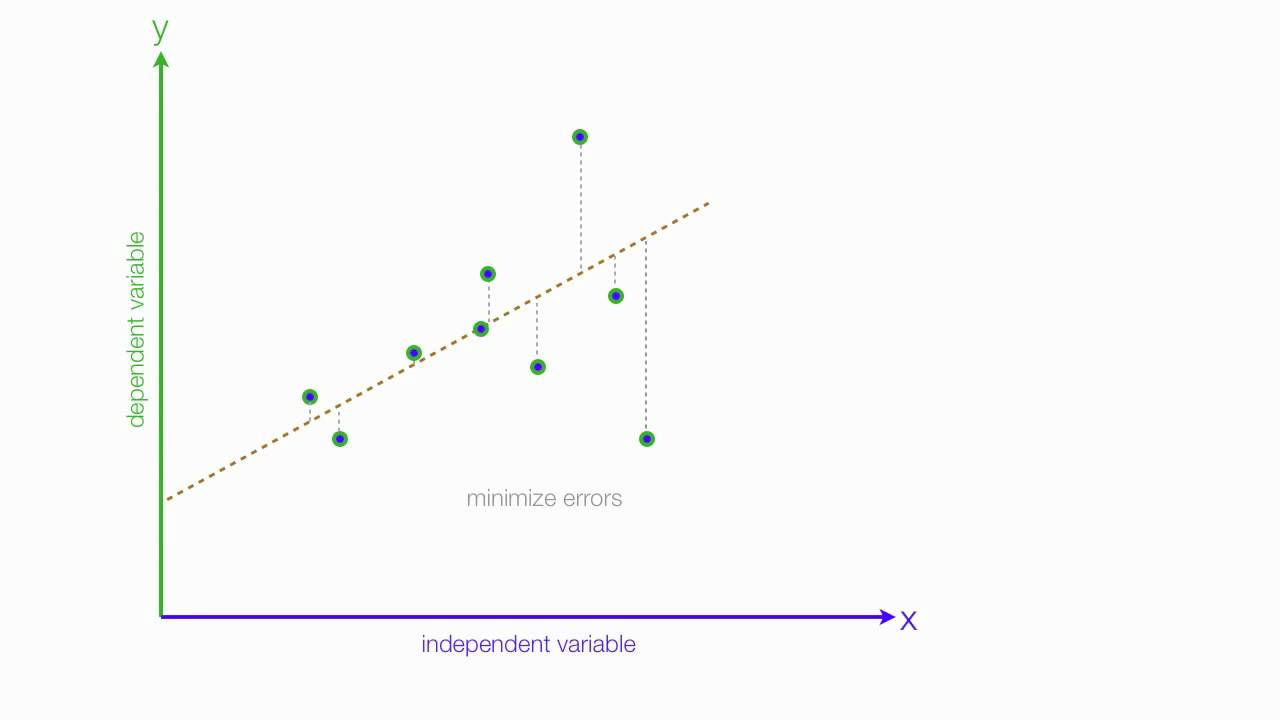Linear regression is a form of regression analysis that involves a dependent variable and only one independent variable. The dotted line that you can see above is the line that fits best through the given set of data points. Its equation is given by the formula:

𝑦=𝜃0+𝜃1𝑥

x">𝑥 is the independent variable

y">𝑦 is the dependent variable

θ0">𝜃0 represents the intercept that the line makes on the y-axis

θ1">𝜃1 represents the slope or gradient of the line

The aim of Linear Regression is to minimize the value of the intercept and the slope in order for the line to best fit the given set of points.

The Mean Square Error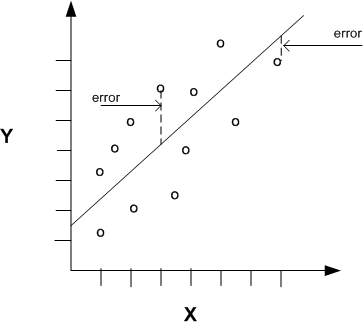The mean square error function is an example of a cost function. We use this to help us minimize the value of our intercept and slope parameters. In order to do this we have to minimize the mean square error, given by the formula: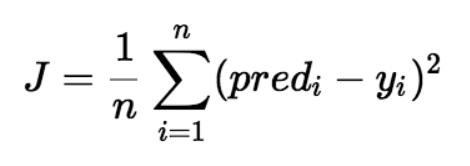The dots represent the actual y value for a particular value of x and we obtain the predicted value of y with the help of the best fit line. In essence what we're doing here is that we first minimize the Mean Square Error and by doing so we find a line that passes through most points reducing the error between our predicted value and our actual value.

Example Using a Program:

We can run linear regression in our model using the scikit-learn module in our program.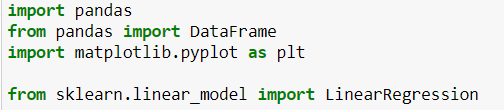In the above bit of program we have imported four modules namely - pandas, matplotlib, LinearRegression from scikit-learn and DataFrame module from pandas.

The data that we are going to use consists of one independent variable and one dependent variable and their actual values can be plotted as: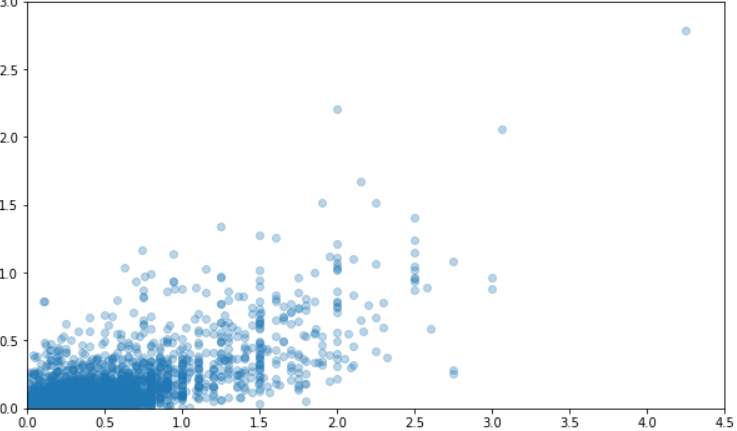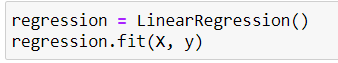We can access the value of our parameters using the code: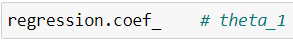This gives us the value of our slope parameter.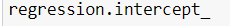This gives us the value of our intercept parameter.

The final step is to plot the line and we obtain the graph as shown below: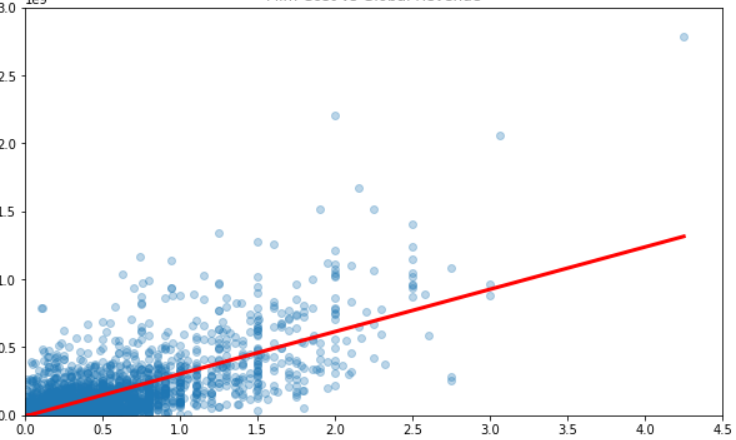𝜃0

𝜃0